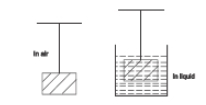#10 | Archimedes Principle:
(Physics) > Mechanical Properties of Fluids
Unable to watch the video, please try another server
Related Practice Questions :

When a body of mass 'm', destiny ${\mathrm{d}}_{\mathrm{B}}$ is suspended from a wire, its elongation is 'e' when the body is in-air. If the body is completely immersed in non-viscous liquid of density d then its elongation is1. $\mathrm{e}\left(1-\frac{{\mathrm{d}}_{\mathcal{l}}}{{\mathrm{d}}_{\mathrm{B}}}\right)$

2. $\mathrm{e}\left(1-\frac{{\mathrm{d}}_{\mathrm{B}}}{{\mathrm{d}}_{\mathcal{l}}}\right)$

3. $\mathrm{e}\left(\frac{{\mathrm{d}}_{\mathrm{B}}}{{\mathrm{d}}_{\mathcal{l}}}-1\right)$

4. $\mathrm{e}\left(\frac{{\mathrm{d}}_{\mathrm{B}}}{{\mathrm{d}}_{\mathcal{l}}}\right)$

High Yielding Test Series + Question Bank - NEET 2020

Difficulty Level:

A sample of metal weighs 210 g in air, 140 g in water and 120 g in an unknown liquid. Then -

1.  The density of the metal is

2.  The density of the metal is

3.  The density of the metal is 4 times the density of unknown liquid

4.  The metal still float in water

High Yielding Test Series + Question Bank - NEET 2020

Difficulty Level:

Two non-mixing liquids of densities $\rho$ and n$\rho$(n>1) are put in a container. The height of each liquid is h. A solid cylinder  floats with its axis vertical and length pL $\left(p<1\right)$ in the denser liquid. The density of the cylinder is d. The cylinder floats with its axis vertical and length pL (p<1) in the denser liquid. The density d is equal to

(a) {2+(n+1)p}$\rho$             (b) {2+(n-1)p}$\rho$

(c) {1+(n-1)p}$\rho$              (d) {1+(n+1)p}$\rho$

High Yielding Test Series + Question Bank - NEET 2020

Difficulty Level:

Two bodies are in equilibrium when suspended in water from the arms of a balance. The mass of one body is 36 g and its density is 9 g / cm3. If the mass of the other is 48 g, its density in g / cm3 is
(a)$\frac{4}{3}$                                         (b) $\frac{3}{2}$
(c) 3                                           (d) 5

An ice berg of density 900 Kg/${\mathrm{m}}^{3}$ is floating in water of density 1000 Kg/${\mathrm{m}}^{3}$. The percentage of volume of ice-cube outside the water is